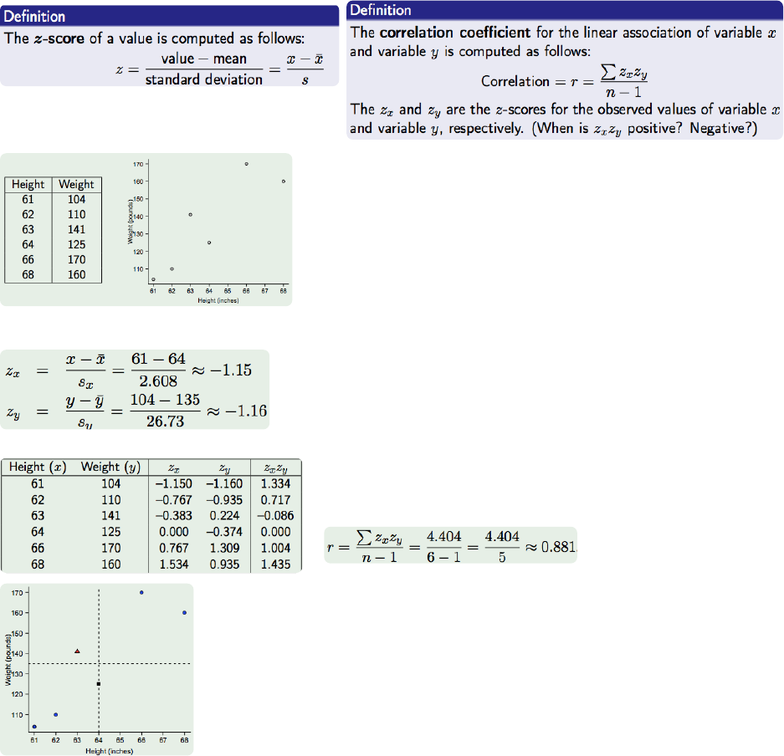Class Notes (1,100,000)
US (490,000)
UCLA (10,000)
STATS (200)
Lecture 24

# STATS 13 Lecture Notes - Lecture 24: Confounding, Dependent And Independent Variables, Standard Deviation

Department
Statistics
Course Code
STATS 13
Professor
Tsiang, Mike
Lecture
24

This preview shows half of the first page. to view the full 3 pages of the document.Review of Causality!
recall from chapter 4!
causality (in statistics)!
treatment variable outcome/response variable!
the causality question: does the treatment variable cause changes in the outcome variable?!
using data from observational studies one conclusions about the association between variables can be made!
causality cannot be concluded from observational studies!
a confounding variable (or confounding factor) is associated with both the explanatory variable and the response
variable that could explain the dierence between the outcomes of the two groups!
!
Correlation Does not imply causation !
correlation is only a measure of linear association. strong correlation suggest that there is a strong linear
relationship between variables!
correlation cannot be used to make statements about causality even if the correlation is close to 1 or -1!
correlation does not imply causation !
!
Formula for the Correlation Coecient!
!
!
Calculating the Correlation Coecient!
!
compute the mean and standard deviation for each variable!
compute the z scores!
!
height variable is x and weight variable is y!
!
for height: x
̄ = 64 and sx= 2.608!
for weight y-bar= 136 and sy = 26.73!
!
then compute zx and zy for each observation!
ex. for a woman who is 61 inches tall and weighs 104 pounds!
!
so the product zxzy for this woman is zx x zy = 1.15 x 1.16 = 1.334!
!
!
!
All z scores and products from the data!
compute Σzxzy by adding the products of the z scores together!
1.334 + .717 - .086 + 0 + 1.004 + 1.435 = 4.404!
!
divide by n-1 to get the correlation coecient !
!
!
!
Points that add to the correlation have values of the two variables that are both
above the mean or both below their means (blue circles)!
Points that subtract from the correlation have the values of one variable above its
mean and the other below its mean (red triangle)!
Points that do not contribute to the correlation have the value of at least one
variable exactly equal to its mean (black square)!
!
!
!
!